# Constant function facts for kids

Kids Encyclopedia Facts

In mathematics, a constant function is a function whose output value is the same for every input value. For example, the function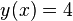$y(x) = 4$ is a constant function because the value of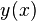$y(x)$  is 4 regardless of the input value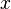$x$ (see image).

## Basic properties

Formally, a constant function f(x):RR has the form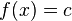$f(x)=c$. Usually we write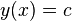$y(x)=c$  or just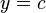$y=c$.

• The function y=c has 2 variables x and у and 1 constant c. (In this form of the function, we do not see x, but it is there.)
• The constant c is a real number. Before working with a linear function, we replace c with an actual number.
• The domain or input of y=c is R. So any real number x can be input. However, the output is always the value c.
• The range of y=c is also R. However, because the output is always the value of c, the codomain is just c.

Example: The function$y(x)=4$  or just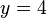$y=4$  is the specific constant function where the output value is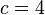$c=4$. The domain is all real numbers ℝ. The codomain is just {4}. Namely, y(0)=4, y(−2.7)=4, y(π)=4,.... No matter what value of x is input, the output is "4".

• The graph of the constant function$y=c$ is a horizontal line in the plane that passes through the point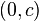$(0,c)$.
• If c≠0, the constant function y=c is a polynomial in one variable x of degree zero.
• The y-intercept of this function is the point (0,c).
• This function has no x-intercept. That is, it has no root or zero. It never crosses the x-axis.
• If c=0, then we have y=0. This is the zero polynomial or the identically zero function. Every real number x is a root. The graph of y=0 is the x-axis in the plane.
• A constant function is an even function so the y-axis is an axis of symmetry for every constant function.

## Derivative of a constant function

In the context where it is defined, the derivative of a function measures the rate of change of function (output) values with respect to change in input values. A constant function does not change, so its derivative is 0. This is often written: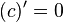$(c)'=0$ .

Example: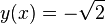$y(x)=-\sqrt{2}$  is a constant function. The derivative of y is the identically zero function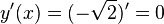$y'(x)=(-\sqrt{2})'=0$  .

The converse (opposite) is also true. That is, if the derivative of a function is zero everywhere, then the function is a constant function.

Mathematically we write these two statements: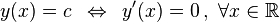$y(x)=c \,\,\, \Leftrightarrow \,\,\, y'(x)=0 \,, \,\,\forall x \in \mathbb{R}$

## Generalization

A function f : AB is a constant function if f(a) = f(b) for every a and b in A.

## Examples

Real-world example: A store where every item is sold for 1 euro. The domain of this function is items in the store. The codomain is 1 euro.

Example: Let f : AB where A={X,Y,Z,W} and B={1,2,3} and f(a)=3 for every aA. Then f is a constant function.

Example: z(x,y)=2 is the constant function from A=ℝ² to B=ℝ where every point (x,y)∈ℝ² is mapped to the value z=2. The graph of this constant function is the horizontal plane (parallel to the x0y plane) in 3-dimensional space that passes through the point (0,0,2).

Example: The polar function ρ(φ)=2.5 is the constant function that maps every angle φ to the radius ρ=2.5. The graph of this function is the circle of radius 2.5 in the plane.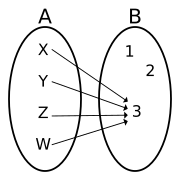Generalized constant function.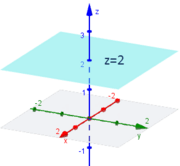Constant function z(x,y)=2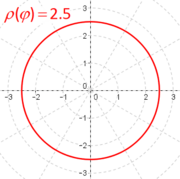Constant polar function ρ(φ)=2.5

## Other properties

There are other properties of constant functions. See on English Wikipedia

## Related pagesIn Spanish: Función constante para niños